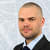iOS Development #5: NumbersJune 24, 2021
Share:

In the previous chapter, we shortly discussed some basic data types, namely numbers, text (string), and logical values (boolean). Let’s take a look at numbers in more details now.

Whole numbers

Int (which we used to declare the age variable) stands for integer. This data type is used to store whole numbers and you can use it to store any whole number between -2 147 483 648 and 2 147 483 647.

Since age can’t be a negative number, we could also use UInt type which stands for unsigned integer and it can store the values from 0 to 4 294 967 295.

Floating-point numbers

If you need to store a floating-point number like 0.5 or 3.1415, Swift offers another two types called Float and Double. While Float has a precision of only 6 decimal digits, Double offers a precision of at least 15 decimal digits. That’s why Double is preferred as it gives you more room for your calculations.

In the US, UK and Australia, the decimal separator is a point (3.1415), in Europe and South America, it’s usually a comma (3,1415) while a point is used as a digit group separator (10.500 means ten thousand and five hundred, not ten point five, zero, zero). This might be quite confusing, so I’ll use a space to separate digit groups (10 500) and points to indicate a floating point.

Arithmetic operators

All programming languages can calculate numbers and Swift is no different. It has several built-in arithmetic operators, that allow us to perform calculations on numbers. Here’s the list of these operators including their respective symbols:

• - subtraction
• * multiplication
• / division
• % modulo (divides and gives the remainder)

Here are few examples:

var score: Int = 0  // initialize score variable with value 0
score = 10 + 20  // score now stores 30
score = score - 20  // 30 stored in score - 20 gives us 10
score = 4 * 2  // score now stores 8
score = 4 / 2  // and now 2
score = 5 % 2  // and now 1

Copy the code above and paste it to your playground. Notice how the 123 symbol appears next to each line of code. When you click on it, it will reveal a pop-up window with Add Viewer button.The number above the button is the result of the operation on that specific line. By clicking the button, you can add the result below the code.Check all results to see if they correspond with values in the comments. This feature can save you time because you don’t need to print(score) to the console each time you change its value.

As you probably remember from your math class, the order of operations can be changed with parentheses. For example, the use of parentheses in score = 4 * (2 + 1) sets score equal to 4 * 3 rather than 8 + 1.

Compound assignment

In the previous examples, you learned that you could update the value of the variable by assigning a completely new value (score = 50) or by modifying the existing value (score = score - 20).

The second case can be shortened by using compound assignment operators so you could write score -= 20 with the same effect. This means that by using this shorthand method, you no longer need to reference score twice.

You can use compound alternative for all five arithmetic operators:

• += add and assign the sum.
• -= subtract and assign the difference.
• *= multiply and assign the product.
• /= divide and assign the quotient.
• %= divide and assign the remainder.

Let’s say you have 15 pears. You gave 5 of them to your friend John and then you gave the half of what’s left to your friend Michal. In the end, all three of you will have the same number of pears.

Now, check how it’s written utilizing the compound assignment:

var myPears: Int = 15
myPears -= 5
myPears /= 2
print(myPears)

Share:Written by

Jan Zavrel

Developer, Author, Teacher, Evernote Certified Consultant.

Related stories

iOS Development #10: If & Else ConditionalsiOS Development #9: Custom TypesiOS Development #8: StringsiOS Development #7: Type Annotation & InferenceiOS Development #6: StructsiOS Development #4: Variables